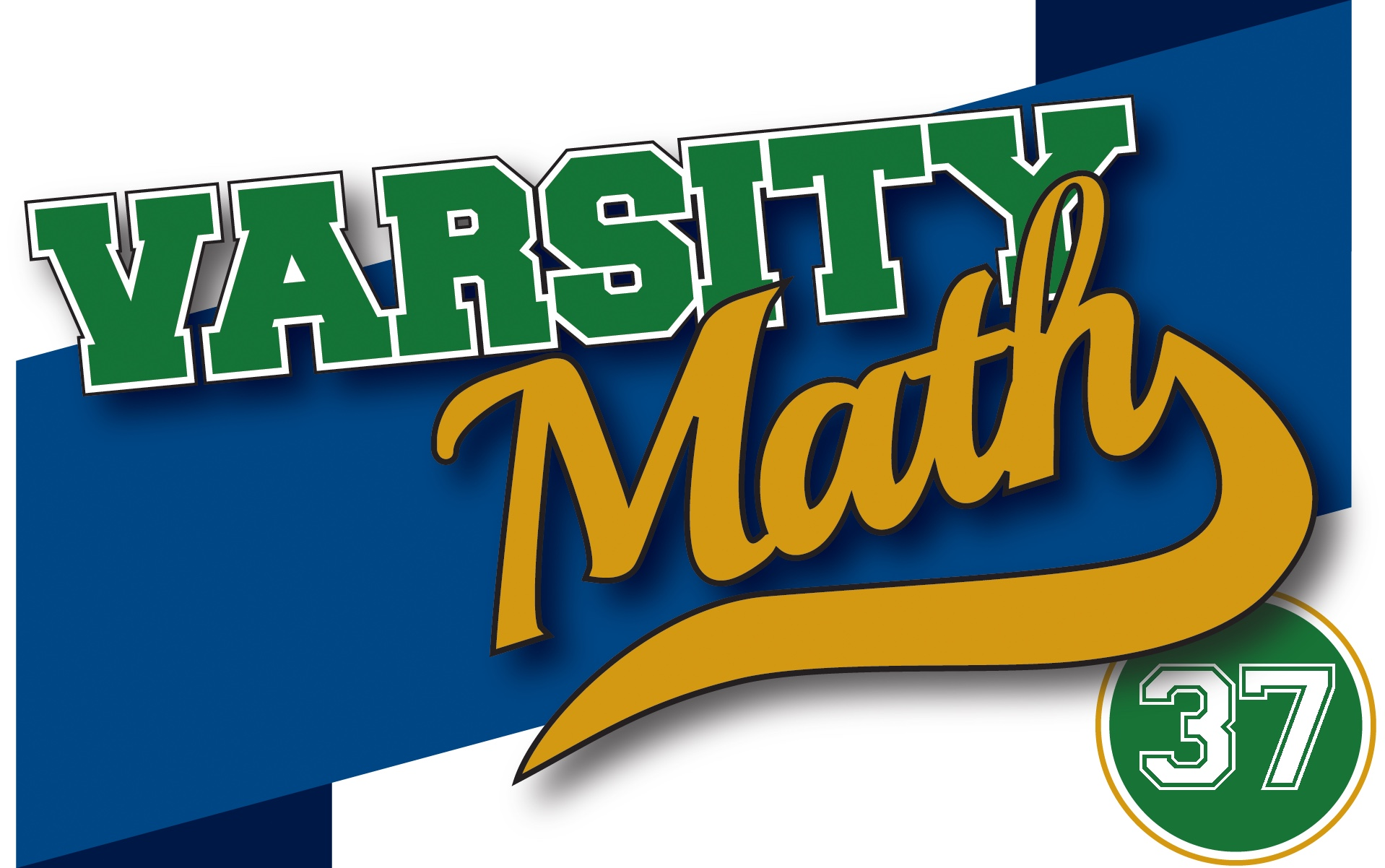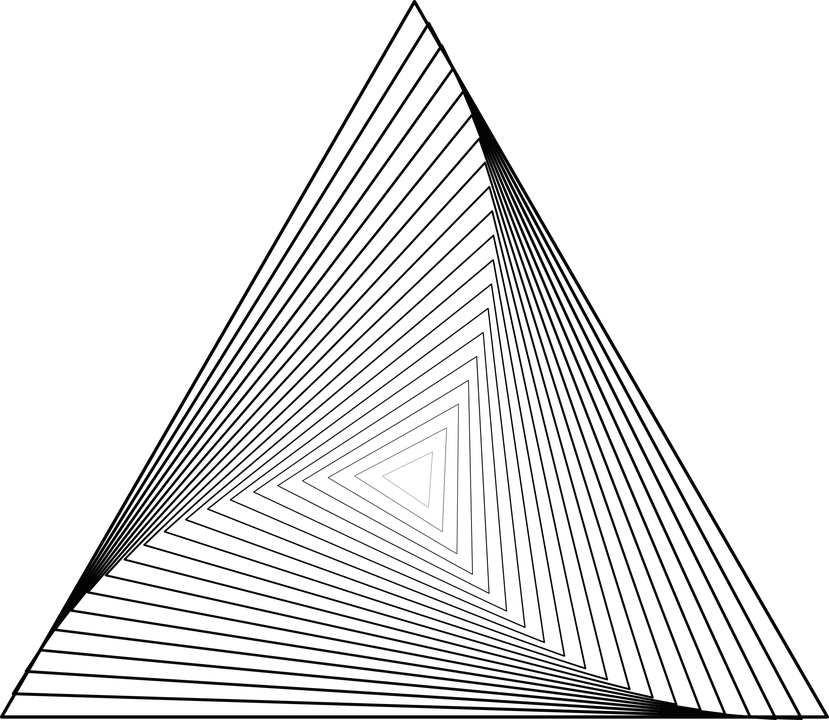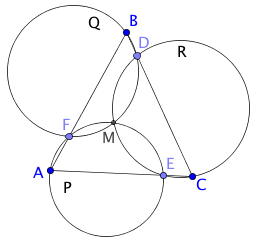The Varsity Math team members liked last week’s problem about a triangle so much that they clamored for more. Coach Newton is all too happy to oblige with two more triangular teasers.### Equal Laterals

T is an equilateral triangle with side length equal to the answer to last week’s Possible Parabolas problem. P is a point in the interior of T such that the distance from P to the farthest vertex of T is twice the distance from P to the nearest vertex of T, and the distance from P to the third vertex of T is 3/2 the distance to the nearest vertex. Let Q, R, and S be the feet of the perpendiculars from P to each of the three sides of T.

What is the sum of the distances PQ + PR + PS?

### Isocircules

Isosceles triangle ABC, with side BC half the length of each of the other two sides, is inscribed in circle Q. Ray r from point A is drawn so that side AC bisects the angle between BA and r. Ray r intersects circle Q again at point D.

What is the ratio of the length BD to BC?

## Solution to week 36

Circle Crossing.Let M be the point of intersection of P and Q other than F; we shall show M also lies on R, establishing the answer as 0. First note that angle FME subtends the arc of a full circle minus the measure of arc FME, which is just twice the measure of the angle at A. In other words, FME subtends an arc of 360 – 2A degrees, and so has measure 180 – A degrees. Similarly, angle DMF has measure 180 – B degrees. Subtracting these two angles from 360 degrees, we see that the measure of angle EMD is A + B degrees. But because the angles of triangle ABC sum to 180 degrees, the measure of angle EMD is therefore 180 – C degrees. But the arc of circle R from E to D clockwise is just the locus of all points which make an angle with E and D of 180 – C degrees, so point M lies on circle R as desired.

## Recent Weeks

Links to all of the puzzles and solutions are on the Complete Varsity Math page.

Come back next week for answers and more puzzles.

[asciimathsf]